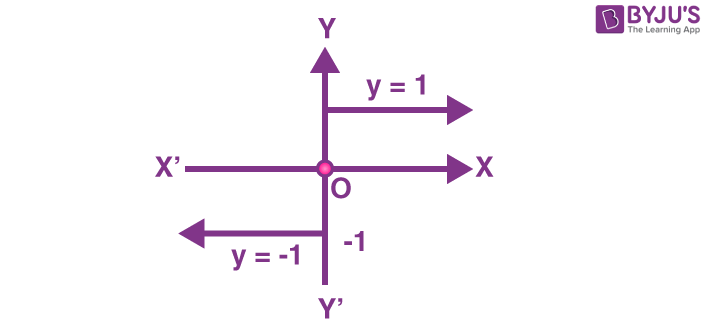# Define signum function draw its graph and find its domain and range

The function f: R —-> R defined by $$f (x) = \left\{\begin{matrix} 1, if x > 0 & & \\ 0, if x = 0 & & \\ -1, if x < 0 & & \end{matrix}\right.$$

is called the signum function or sign function. The graph of the signum function is given belowDomain = R

Range = {-1, 0, 1}